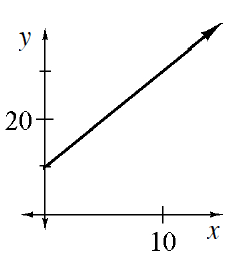### Home > INT3 > Chapter 1 > Lesson 1.1.2 > Problem1-16

1-16.

Junior is saving money in his piggy bank. He starts with $10$ cents and adds two pennies each day. Create a table, draw a complete graph, and write an equation to model this situation.

Make a table where $x$ represents the number of days and $y$ represents the total so far.

How much did he start with on day $0$?

$10$ cents.

How much did he have at the end of day $1?$ Day $2$$?$...

$12$, $14$, ...

What is the $y$-intercept? The slope?

$(0,10)$ and $m=2$Completed the table in the eTool below to graph the function.
Click the link to the right for full version of the eTool: Int3 1-16 HW eTool